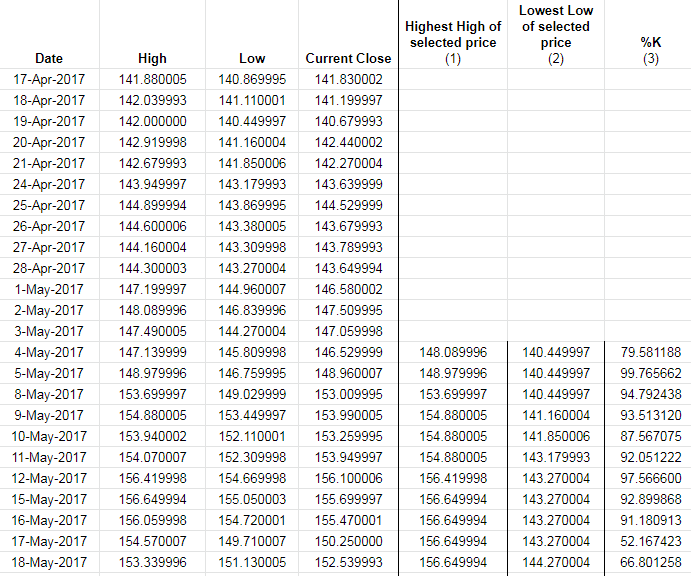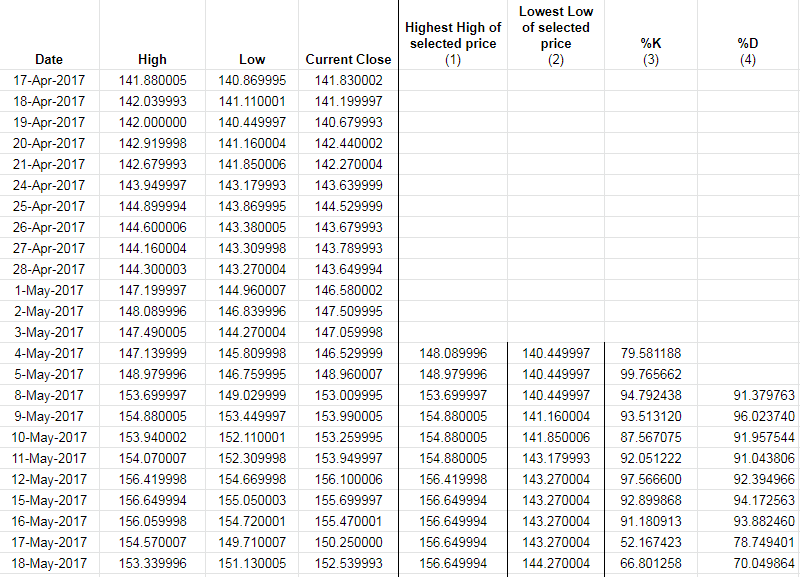# Main indicators

Please purchase the course before starting the lesson.

by Masswaveindia

MOVING AVERAGES

Moving averages are one of the easiest indicators to learn and understand . However their correct use is often neglected due to not comprehending the fact of sustained rhythms, phases and cycles in the markets. One cannot apply a certain moving average all the time, for that will be denying the existence of the various forces (which we will discuss in the master class) within the markets.

Anything simple as it may be, if it is widely used , it becomes an excellent tool in the hands of a knowledgeable trader. It has been noticed time and again that the markets react to the 50 day and 200 day period(notice I have not used the word ‘day’ here and mentioned period only) This phenomenon  will further become clear as we move on to the trading and the master class.

Moving averages also are the building blocks for one of the important indicators like MACD , Rsi etc.

Moving averages can be simple or weighted.

SIMPLE MOVING AVERAGE

Most moving averages are based on the closing prices and are simply the average price of a security over a specific number of periods. For example a simple 5 day moving average is the recurring average of the last 5 periods.

Example   Let us say a stock price moves on a daily basis as follows

Daily closing prices  10 11 12 13 14 15 16 17 18 19 20

Now the 5 day moving average on the 1st day will be 10 +11+12+13+14/5 = 12

The second day of the moving average drops the 1st day and takes the average of the next 5 days or

11+12+13+14+15 /5=13

and so on.

Exponential moving average is similar to simple moving average but gives more weightage to the most recent. For uniformity , I have found the use of simple moving average much easier and practical.

Now the question arises as to which moving average to use at what time. The most popular moving averages are the 20 day, 50 day and the 200 day moving averages. Will touch upon how to trade using indicators only  at a later stage.

HOW TO USE THE MOVING AVERAGES

It is very important to understand that moving average used in isolation can prove to be counterproductive. A stock can move AROUND the particular moving average line multiple times giving whipsaws(losing money on every small rise and fall).

Therefore a moving average must be used only n conjunction with other forms of technical analysis.

Below is an example of the 50 and the 200 day moving average for the nifty index. on a daily chart.I have marked the supports or touch points where the nifty rose from the 50 day  or the 200 day moving average.

Similarly one can use the 9 day or the 20 day moving average also.

THE 50 DAY AND THE 200 PERIOD  MOVING AVERAGE WORK WELL IN ALL PERIODS.

Above is a very important point for consideration. Notice I have used the word period and not the day in fron to 50 and 200.

This will become evident in below chart of different duration (5 MINS CHART)In the 5 minute chart you have the 50 period (blue) and the 200 period (red) moving average. The points of support and resistance are highlighted on the chart.

So the question will arise which moving average to use . The answer is ‘it is not fixed but is a variable depending on the stock and its volatility. This will be shown by live examples in later classes.

Excercise

To find the supports and resistance in the NIFTY chart on the 50 and 200 period at different time frames.

RSI

This stands for ‘Relative Strength Index. ‘ It is one of the very widely used indicator specially for overbought and oversold conditions.

RSI was invented/developed by J.Welles Wilder. This is a momentum oscillator. It measures the speed and change of price action. RSI will always fluctuate between 0 and 100. According to the standard form, RSI is considered overbought when above 70 and oversold when below 30. RSI can be seen in different time frames.

Key factor of RSI is divergence which can AT TIMES give a warning about the impending fall. However it must be noted that RSI can be overbought and show divergence over a long period without affecting the markets. So it signals can be taken as warning signs rather than absolute condition.

Nowadays , with the advent of computers and software, calculation is done automatically and you have a ready made oscillator , which you can observe with a flick of the keyboard. However giving below the calculations for academic interest and a deeper understanding of the concept.

Calculation of RSI

RSI =          100         –        100/1+RS

RS= Average gain/Average loss  over the period chosen. Now the default period chosen by Wilder is 14

Following are the key steps in calculation of RSI

Step 1       Calculate the First average gain (sum of gains over past 14 periods/14)

Similarly calculate the First average loss (Sum of losses over past 14 periods/14)

Subsequent calculations as follows

Average Gain =(average previous gain ) x 13   + current gain/14

Average Loss  =(Average previous loss) x 13 +current loss /14

Actually converting RS into RSI is a clever way of making the indicator into a oscillator between 0 and 100

DIVERGENCE

This takes place when there is a rise or fall in price which is not followed up by the rise or fall  in RSI in the same proportion. example of same is given below in the chart of SBIExcercise

Mark all divergences on the charts of SBI , Tata Motors and Reliance

RSI overbought and oversold.

When RSI crosses above 70 it is said to be overbought and below 70 oversold.

This is evident from the chart of SBI below.Excercise

Mark all overbought and oversold points on the charts of SBI. Tata Motors and Reliance on daily and weekly charts.

STOCHASTIC OSCILLATOR

The stochastic oscillator shows the present price in context of the extreme ranges of price over a given time. The time period by default is 14 days. It is expressed in percentage and calculated as follows

Current closing price – lowest low price (over the chosen time period)/highest high price -lowest low price (over the selected range ) x 100

Method of using

This is never used in isolation but used along with RSI and MACD. More about this at the end of the chapter.

Calculations

We will use the example of APPLE prices over a period of time

The accepted range is usually 14 days. We will calculate %K by using the information on the stock of Apple in the month of April-May 17.

Thus, the table would be as follows,Explaining the market example

To explain this, let’s take the example of %K for the row with date 4 May 2017.

1. To select the Highest high of selected price (1), we check the dates from 17 April to 4 May of the column, “High”, which is 148.089996
2. For Lowest Low of selected price (2), the value is 140.449997
3. To calculate the %K, the formula is,

[(Current closing price) – (lowest low price in selected range)] * 100
[highest high price in selected range) – (lowest low price in selected range)]

= [146.529999 – 140.449997] / [148.089996 – 140.449997] * 100
= (6.080002 / 7.639999) * 100
= 0.7958118 *100
=79.58118%

Similarly, we fill in the rest of the fields as well.

4. %D is simply the 3-day simple moving average (SMA) of the %K.

Thus, the table will be updated as follows,Back to: Technical Analysis Course